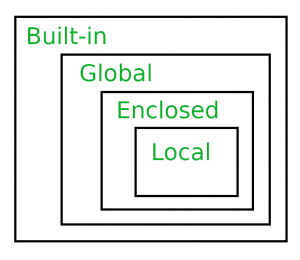# Scope Resolution in Python | LEGB Rule

Namespaces : A namespace is a container where names are mapped to objects, they are used to avoid confusions in cases where same names exist in different namespaces. They are created by modules, functions, classes etc.

Scope : A scope defines the hierarchical order in which the namespaces have to be searched in order to obtain the mappings of name-to-object(variables). It is a context in which variables exist and from which they are referenced. It defines the accessibility and the lifetime of a variable. Let us take a simple example as shown below:

 `pi ``=` `'outer pi variable'` ` `  `def` `print_pi(): ` `    ``pi ``=` `'inner pi variable'` `    ``print``(pi) ` ` `  `print_pi() ` `print``(pi) `

Output:

```inner pi variable
outer pi variable
```

Above program give different outputs because the same variable name pi resides in different namespaces, one inside the function print_pi and the other in the upper level. When print_pi() gets executed, ‘inner pi variable‘ is printed as that is pi value inside the function namespace. The value ‘outer pi variable‘ is printed when pi is referenced in the outer namespace. From the above example, we can guess that there definitely is a rule which is followed, in order in decide from which namespace a variable has to be picked.

Scope resolution via LEGB rule :
In Python, the LEGB rule is used to decide the order in which the namespaces are to be searched for scope resolution.
The scopes are listed below in terms of hierarchy(highest to lowest/narrowest to broadest):

• Local(L): Defined inside function/class
• Enclosed(E): Defined inside enclosing functions(Nested function concept)
• Global(G): Defined at the uppermost level
• Built-in(B): Reserved names in Python builtin modulesLocal Scope :
Local scope refers to variables defined in current function.Always, a function will first look up for a variable name in its local scope. Only if it does not find it there, the outer scopes are checked.

 `# Local Scope ` ` `  `pi ``=` `'global pi variable'` `def` `inner(): ` `    ``pi ``=` `'inner pi variable'` `    ``print``(pi) ` ` `  `inner() `

Output:

```inner pi variable
```

On running the above program, the execution of the inner function prints the value of its local(highest priority in LEGB rule) variable pi because it is defined and available in the local scope.

Local and Global Scopes :
If a variable is not defined in local scope, then, it is checked for in the higher scope, in this case, the global scope.

 `# Global Scope ` ` `  `pi ``=` `'global pi variable'` `def` `inner(): ` `    ``pi ``=` `'inner pi variable'` `    ``print``(pi) ` ` `  `inner() ` `print``(pi) `

Output:

```inner pi variable
global pi variable
```

Therefore, as expected the program prints out the value in the local scope on execution of inner(). It is because it is defined inside the function and that is the first place where the variable is looked up. The pi value in global scope is printed on execution of print(pi) on line 9.

Local, Enclosed and Global Scopes :
For the enclosed scope, we need to define an outer function enclosing the inner function, comment out the local pi variable of inner function and refer to pi using the nonlocal keyword.

 `# Enclosed Scope ` ` `  `pi ``=` `'global pi variable'` ` `  `def` `outer(): ` `    ``pi ``=` `'outer pi variable'` `    ``def` `inner(): ` `        ``# pi = 'inner pi variable' ` `        ``nonlocal pi ` `        ``print``(pi) ` `    ``inner() ` ` `  `outer() ` `print``(pi) `

Output:

```outer pi variable
global pi variable
```

When outer() is executed, inner() and consequently the print functions are executed, which print the value the enclosed pi variable. The statement in line 10 looks for variable in local scope of inner, but does not find it there. Since pi is referred with the nonlocal keyword, it means that pi needs to be accessed from the outer function(i.e the outer scope). To summarize, the pi variable is not found in local scope, so the higher scopes are looked up. It is found in both enclosed and global scopes. But as per the LEGB hierarchy, the enclosed scope variable is considered even though we have one defined in the global scope.

Local,Enclosed,Global and Built-in Scopes :
The final check can be done by importing pi from math module and commenting the global, enclosed and local pi variables as shown below:

 `# Built-in Scope ` `from` `math ``import` `pi ` ` `  `# pi = 'global pi variable' ` ` `  `def` `outer(): ` `    ``# pi = 'outer pi variable' ` `    ``def` `inner(): ` `        ``# pi = 'inner pi variable' ` `        ``print``(pi) ` `    ``inner() ` ` `  `outer() `

Output:

```3.141592653589793
```

Since, pi is not defined in either local, enclosed or global scope, the built-in scope is looked up i.e the pi value imported from math module. Since the program is able to find the value of pi in the outermost scope, the following output is obtained,

My Personal Notes arrow_drop_upCheck out this Author's contributed articles.

If you like GeeksforGeeks and would like to contribute, you can also write an article using contribute.geeksforgeeks.org or mail your article to contribute@geeksforgeeks.org. See your article appearing on the GeeksforGeeks main page and help other Geeks.

Please Improve this article if you find anything incorrect by clicking on the "Improve Article" button below.

Article Tags :

6

Please write to us at contribute@geeksforgeeks.org to report any issue with the above content.